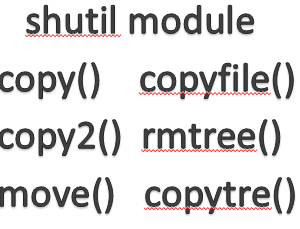## Python Pattern Based ProgramAs we are already aware of pattern programs as we are doing this from basic of programming , still it places huge impact on coding.This also creates an environment where the coder needs to think about sequence of the objects and then iterating in loops will make coding entertaining. So here are some of the pattern programs which will be useful for you.

1.Write a program to print the following patterns.

```for i in range(1, 6):
print('* '*(i))```

```*
* *
* * *
* * * *
* * * * * ```

2.Write a program to print the following patterns.

```n=5
for i in range(0,n):
for j in range(i):
print('* ',end='')
print()

*
**
***
****
```

3.Write a program to print the following patterns.

```for j in range(6,0,-1):
print('* '*(j))

* * * * * *
* * * * *
* * * *
* * *
* *
*
```

4.Write a program to print the following patterns.

```n=5
for x in range(n,0,-1):
for y in range(x):
print('* ',end='')
print()

* * * * *
* * * *
* * *
* *
*
```

5.Write a program to print the following patterns.

```n=5
for i in range(0,n):
for j in range(i):
print('* ',end='')
print()

n=5
for x in range(n,0,-1):
for y in range(x):
print('* ',end='')
print()

*
* *
* * *
* * * *
* * * * *
* * * *
* * *
* *
* ```

6. Write a program to print the following patterns.

```for i in range(1, 6):
print('* '*(i))

for j in range(6,0,-1):
print('* '*(j))*
* *
* * *
* * * *
* * * * *
* * * * * *
* * * * *
* * * *
* * *
* *
*
```

7.Write a program to print the following patterns.

```n=5
for i in range(0,5):
print(' *'*n) * * * * *
* * * * *
* * * * *
* * * * *
* * * * *
```

Category:Hi, I’m Steven, a Florida native, who left my career in corporate wealth management six years ago to embark on a summer of soul searching that would change the course of my life forever.

• ## The term ‘npm’ is not recognized as the name of a cmdlet, function, script file, or operable program• ## Exploring Essential File Operations in Python with shutil library